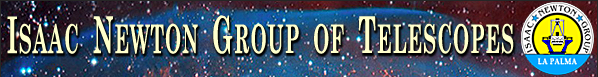Home > Astronomy > INGRID > Sensitivity

## Sensitivity

The "limiting magnitude" of an IR instrument can be easily predicted when the observations are taken in the background-limited case (i.e. the readout noise is negligible compared to the background shot noise).

From the zeropoints and the background brightnesses in J, H and Ks, we have calculated the expected limiting magnitude as a function of: (i) the exposure time, (ii) the seeing (for point sources) and (iii) the required signal to noise ratio. You should bear in mind that the background brightness is highly variable, so that the calculated limiting magnitude can vary from night to night - see the plots of limiting magnitudes for point sources and extended sources.

The limiting magnitudes have been calculated using the equation (McLean 1997)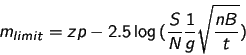This equation assumes that observations are taken in the background limited case, whereis the predicted limiting magnitude.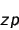is the zeropoint of the instrumental scale (i.e. the magnitude of a source that gives a flux of 1 ADU/s).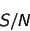is the signal to noise ratio for the detection of the limiting magnitude.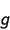is the gain (in e-/ADU) of the detector.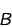is the background signal per pixel (in e-/s/pix).is the number of pixels covered by a point source. We assume that n=pi*(seeing/0.238)2 ie. the source radius is equal to the seeing (fwhm), which is probably pessimistic. For extended objects, the limiting magnitude per arcsec2 has been given instead i.e. n=1/0.2382.is the total on-object integration time (ie. the number of object frames × exposure time of each individual frame).

From the formula above, it can be seen that if the background increases by a factor of 2, the limiting magnitude in the plots would decrease by ~0.38 mag (or ~0.38 mag/arcsec² for extended objects). In order to reach the same limiting magnitude, one would need to increase the total on-object exposure time by a factor of 2.

If the seeing were to deteriorate by a factor of 2, the limiting magnitudes in the plots would decrease by ~0.75 mag. Consequently, the total on-object exposure time would have to be increased by a factor of 4 to reach the same limiting magnitude.

If a S/N of 5 (instead of 3) were required, the limiting magnitudes in the plots would decrease by ~0.55 mag. In order to reach the same limiting magnitude, one would need to increase the total on-object exposure time by a factor of ~2.8.

Note that you can also use the exposure time calculator SIGNAL to calculate the number of photons (from both the object and the sky) that will be detected during an INGRID exposure of a point or extended source.

 Top | BackContact:  (INGRID Instrument Specialist)Last modified: 17 June 2012